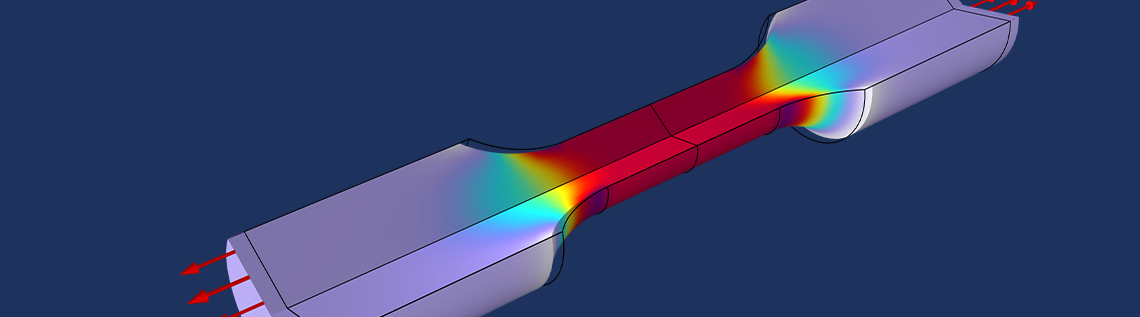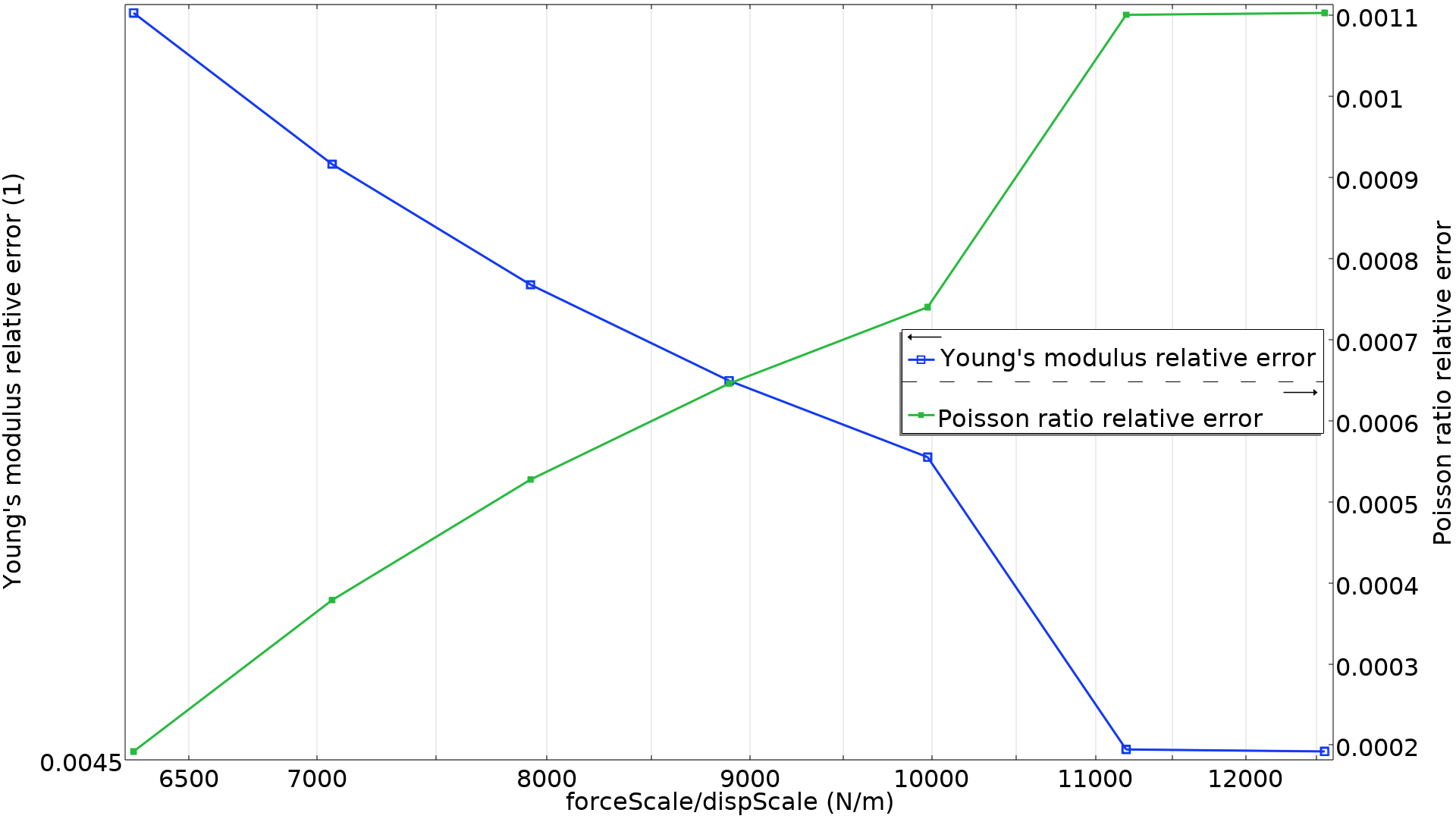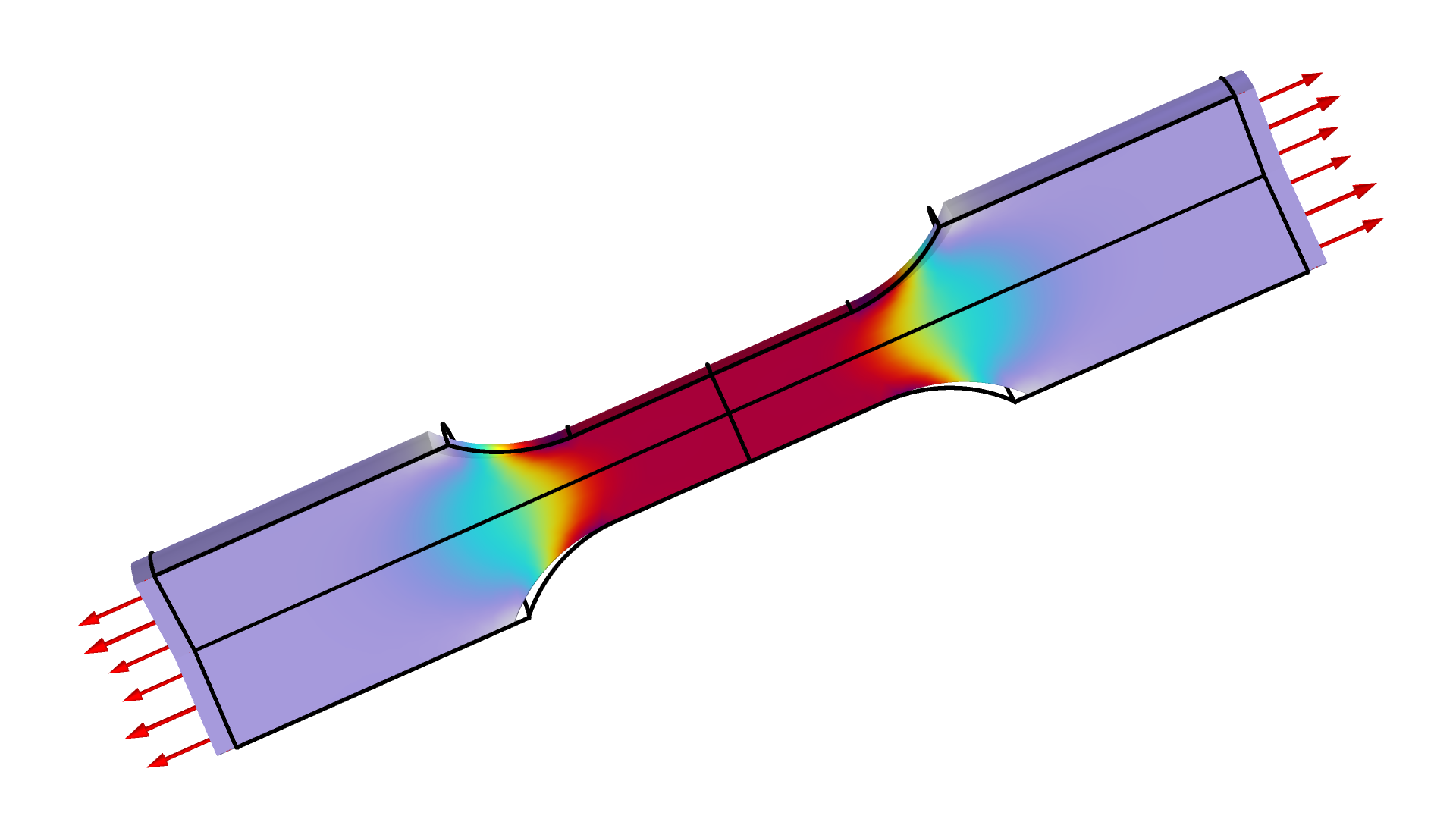# 如何在 COMSOL® 中使用最大似然法进行参数估计

2022年 5月 13日

### 使用最小二乘法避免手动调整### 数据采样时的概率和统计

P=\int_a^bf(x)dx.

P=f(x)dx.### 最小二乘目标和最大似然参数估计

g(x-x^e,\sigma)=\frac{1}{\sigma\sqrt{2\pi}}e^{-\frac{(x-x^e)^2}{2\sigma^2}},

P=\prod_i^n g(x_i,x_i^e,\sigma) = \prod_i^n \frac{1}{\sigma\sqrt{2\pi}}e^{-\frac{(x_i-x_i^e)^2}{2\sigma^2}}.

\log(P) &=& \sum_i^n \log\left(\frac{1}{\sigma\sqrt{2\pi}}e^{-\frac{(x_i-x_i^e)^2}{2\sigma^2}}\right) \\
&=& \sum_i^n\left( -\frac{(x_i-x_i^e)^2}{2\sigma^2}-\log\left(\sigma\sqrt{2\pi}\right)\right)\Leftrightarrow \\
-\log(P) &=& n\log\left(\sigma\sqrt{2\pi}\right) + \frac{_1}{^2}\sum_i^n\frac{(x_i-x_i^e)^2}{\sigma^2}.

### 拉伸试验的最大可能性P &=& P_F P_r \Leftrightarrow -\log(P)=-\log(P_f)-\log(P_r).

COMSOL Multiphysics 内置的最小二乘法目标法，让使用自定义目标来解决最大似然参数估计问题变得相当简单。COMSOL 案例库中提供的使用最大似然法进行参数估计模型，通过添加正态分布的噪点数据来生成合成数据。该模型在这些数据的基础上恢复材料参数和标准差，由此产生的力和径向位移如下图所示。